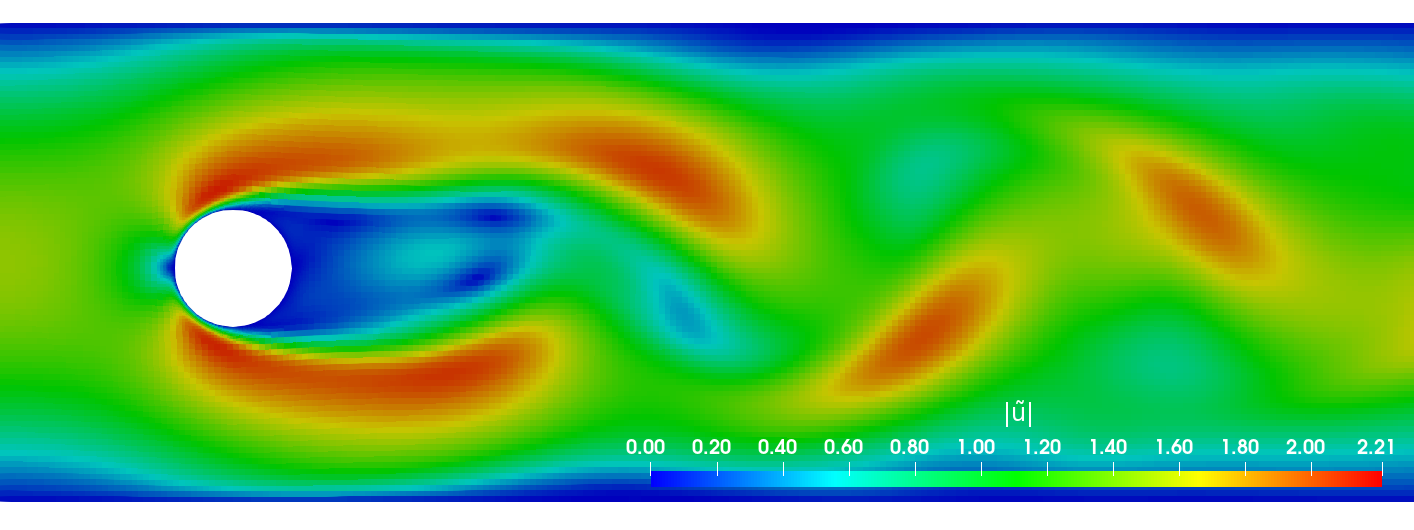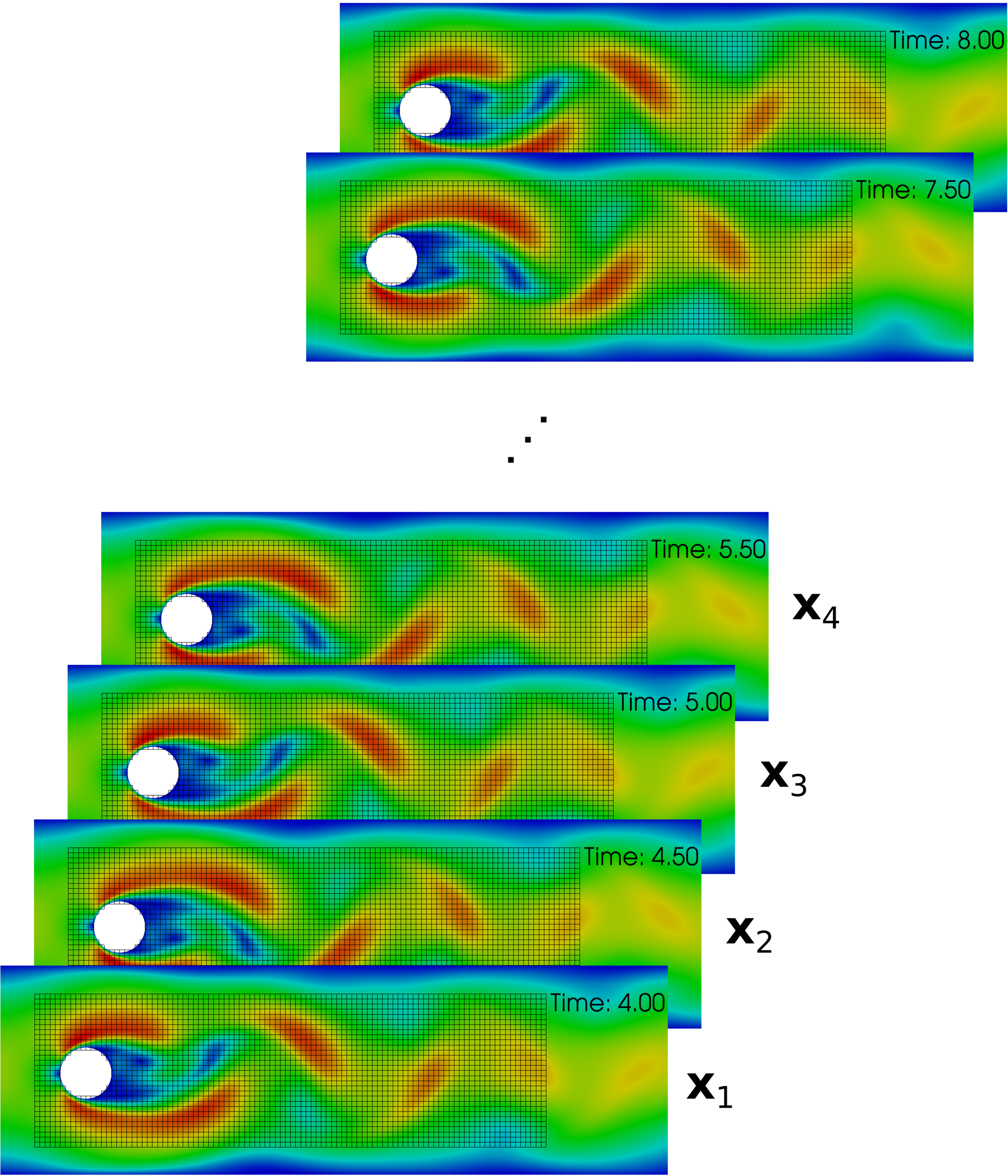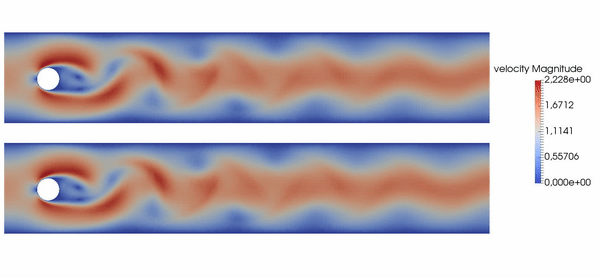### Creating data-driven CFD workflows using OpenFOAM and PyTorch

Andre Weiner1, Chiara Pesci2, Tomislav Marić3, Richard Semaan1, Dieter Bothe3
1TU Braunschweig ISM, 2 ESI GmbH, 3 TU Darmstadt MMA

andreweiner.github.io/reveal.js/of_conf_2020.html#/

## Outline

1. Data-driven workflows
2. PyTorch
3. Supervised learning
4. Unsupervised learning
5. Reinforcement learning

## What is data?primary data: scalar/vector fields, boundary fields, integral values


# log.rhoPimpleFoam
Courant Number mean: 0.020065182 max: 0.77497916
deltaT = 6.4813615e-07
Time = 1.22219e-06

PIMPLE: iteration 1
diagonal:  Solving for rho, Initial residual = 0, Final residual = 0, No Iterations 0
DILUPBiCGStab:  Solving for Ux, Initial residual = 0.0034181127, Final residual = 6.0056507e-05, No Iterations 1
DILUPBiCGStab:  Solving for Uy, Initial residual = 0.0052004883, Final residual = 0.00012352706, No Iterations 1
DILUPBiCGStab:  Solving for e, Initial residual = 0.06200185, Final residual = 0.0014223046, No Iterations 1
limitTemperature limitT Lower limited 0 (0%) of cells
limitTemperature limitT Upper limited 0 (0%) of cells
limitTemperature limitT Unlimited Tmax 329.54945
Unlimited Tmin 280.90821


Checking geometry...
...
Mesh has 2 solution (non-empty) directions (1 1 0)
All edges aligned with or perpendicular to non-empty directions.
Boundary openness (1.4469362e-19 3.3639901e-21 -2.058499e-13) OK.
Max cell openness = 2.4668495e-16 OK.
Max aspect ratio = 3.0216602 OK.
Minimum face area = 7.0705331e-08. Maximum face area = 0.00033983685.  Face area magnitudes OK.
Min volume = 1.2975842e-10. Max volume = 6.2366859e-07.  Total volume = 0.0017254212.  Cell volumes OK.
Mesh non-orthogonality Max: 60.489216 average: 4.0292071
Non-orthogonality check OK.
Face pyramids OK.
Max skewness = 1.1453509 OK.
Coupled point location match (average 0) OK.



secondary data: log files, input dictionaries, mesh quality metrics, ...

## What is a data-driven workflow?Example: creating a surrogate or reduced-order model based on numerical data.Example: creating a space and time dependent boundary condition based on numerical or experimental data.Example: creating closure models based on numerical data.Example: active flow control or shape optimization.

## ML/deep learning frameworks

criteria: workflow, algorithms, data size, programming language, platform, ...

Why PyTorch?

• easy to use and set up
• Python/C++/Java frontend
• easy exchange and optimization of models thanks to TorchScript
• Python and C++ APIs closely aligned

## Getting started

### Supervised learningCreating a mapping from features to labels based on examples.

Example: mapping the velocity from two-phase to single-phase simulations.

Extension to dynamically deforming bubbles together with Irian Hierck, Claire Claassen, and Maike Baltussen @ TU Eindhoven

• Data:
1. rise velocity
2. interface position
3. interface velocity
• PyTorch models:
1. inlet velocity $\mathbf{u}_{in} = \mathbf{u}_{in}(\tilde{t})$
2. bubble radius $r_{b} = r_{b}(\tilde{t}, \vartheta)$
3. surface velocity $\mathbf{u}_{\Sigma} = \mathbf{u}_{\Sigma}(\tilde{t}, \vartheta)$
• OpenFOAM:
• boundary conditions for velocity and mesh motion

Transient concentration field for different Reynolds numbers $Re$ and constant Schmidt number $Sc=100$.

Mesh motion and zoom view of concentration boundary layer for $Re=569$ and $Sc=100$.Global Sherwood number $Sh$ for two different mesh resolutions (3250 and 6500 cells/diameter). ~7h, serial, 2.4 GHz

## Unsupervised learningFinding patterns in unlabeled data.

Example: dynamic mode decomposition (DMD) of the flow past a cylinder.## Classical DMD

• data matrices: $$\mathbf{X} = \left[ \mathbf{x}_1, \mathbf{x}_2, \dots, \mathbf{x}_{N-1} \right]^T\text{, }\mathbf{X}^\prime = \left[ \mathbf{x}_2, \mathbf{x}_3, \dots, \mathbf{x}_{N} \right]^T$$
• singular value decomposition (SVD): $$\mathbf{X} = \mathbf{U}\mathbf{\Sigma} \mathbf{V}^T$$
• rank $r$ approximation of linear operator: $$\mathbf{A}=\mathbf{X}^\prime \mathbf{X}^{-1} \text{, } \tilde{\mathbf{A}} = \mathbf{U}^T_r \mathbf{A}\mathbf{U}_r$$
• Eigen-decomposition: $$\tilde{\mathbf{A}}\mathbf{W} = \mathbf{W}\mathbf{\Lambda}\text{, } \Phi = \mathbf{X}^\prime \mathbf{V} \mathbf{\Sigma}^{-1}\mathbf{W}$$

## DMD in OpenFOAM

• implemented as function object STDMD (streaming DMD)
• main output:
• temporal behavior: STDMD.dat in postProcessing
• spatial modes: modeReal1U in time folders
• What is this useful for?
• reconstruct fields at any time $t$
• find and analyze coherent structures
• build reduced-order models (ROMs)## (Deep) Reinforcement learningCreate an intelligent agent that learns to map states to actions such that cumulative rewards are maximized.

Example: active flow control for drag reduction.Source: Jean Rabault et al., check out the code! Follow our progress on Github; together with Darshan Thummar.

Example: accelerating OpenFOAM simulations.